Curated Data Sets

Although you’ve made a good start at writing your own genome annotation program, as you might expect, a number of useful genome annotation resources already exist for the yeast genome. Have a look at the data sets available on the yeast genome website under Curated Data.

Files ending in “.tab” are “tab” (or “”) delimited and can be easily loaded in R using the read.delim function.

Here are two data sets of particular note:

• SGD_features.tab: A more comprehensive version of the feature annotation table you’ve been working on building.

• go_slim_mapping.tab: A currated set of “Gene Ontology” (GO) terms, describing the process, function and localization of each gene product encoded in the yeast genome. The “slim” data set maps each protein/function RNA to a single “term”; the fill GO go mapping dataset allows single proteins/RNAs to be mapped to multiple terms.

Goal: Ask a question, answer it with a picture!

Your goal for the rest of lab today is to ask an interesting question about the yeast genome that can be answered by analyzing the data you find in one or more of the curated data sets on SGD. By the end of lab try to have one data visualization (or more) that answer’s that question!

Some ideas:

• What is the distribution of gene or protein sizes across the yeast genome? Of introns? Of functional RNAs?
• Are there correlations between the processes proteins are involved in and their size? Or location within the genome?
• Are there biases in which genes contain introns?
• Do short chromosomes have more or longer introns? [credit to Joseph]

In the section below I’ll introduce a very powerful graphis package for R that you will probably want to use to explore different visualization strategies.

Grammar of Graphics in R (ggplot2)

Although the base graphics capabilities provided by R are extremely flexible and powerful, the defaults visual paramters and programming interface are pretty ugly and outdated. Hadley Wickham’s ggplot2 package, inspired by Leland Wilkinson’s call for a grammer of graphics, is a far better easier platform to work with and is a better choice new commers to R.

So today, we’ll focus on introducing plotting in R using the ggplot2 package instead of the base plot(...) function. Hopefully you’ll be excited by how easy it is to create some stunning plots!

ggplot2

If it isn’t already available, you can install the ggplot2 package by running install.packages("ggplot2"). Once the package is installed, loading ggplot2 for use in a script or at the console can be done using the library function:

library(ggplot2)

Here, I’ll demonstrate some example uses of ggplot2, but for many more ideas of what you can do with the library see the full documentation: docs.ggplot2.org.

For these examples we’ll use the built in diamonds dataset. See ?diamonds for detailed information about this sample data.

Constructing a plot

The general workflow for constructing plots using ggplot2 will be to first describe the structure of the data that you want to visualize and then apply visualization functions to that structure. Although this coding idiom, and the syntax that has been designed to support it, might feel strange at first you will soon come to appreciate the elegance of separating your description of what data you want to visualize from the particulars of how you want to draw that visualization. This separation will feel very strange at first if you are coming from other plotting/graphing platforms which generally conflate these two concerns. However, as you walk through the following examples hopefully the power and flexibility of this design pattern will become clear!

The first step in producing a ggplot2 visualization is to initialize a data structure that describes the (1) source of the data we want to work with and (2) the relationship between variables that we want to visualize (this is called the “aesthetic mapping” in ggplot lingo):

# Here's the structure of the diamonds dataset
##   carat       cut color clarity depth table price    x    y    z
## 1  0.23     Ideal     E     SI2  61.5    55   326 3.95 3.98 2.43
## 2  0.21   Premium     E     SI1  59.8    61   326 3.89 3.84 2.31
## 3  0.23      Good     E     VS1  56.9    65   327 4.05 4.07 2.31
## 4  0.29   Premium     I     VS2  62.4    58   334 4.20 4.23 2.63
## 5  0.31      Good     J     SI2  63.3    58   335 4.34 4.35 2.75
## 6  0.24 Very Good     J    VVS2  62.8    57   336 3.94 3.96 2.48
# Construct a ggplot object to visualize the relationship between clarity and depth
p <- ggplot(diamonds, aes(clarity, depth))

The aes function in the above block sets up the structure of our visualization: we’re interested in considering depth (quantitative) as a function of clarity (qualitative). The p object doesn’t yet hold any information about how this relationship should be visualized, only the structure of what is being visualized.

You can use the + operator to add visualization functions to this underlying structure to actually produce plots. The data projection functions all start with geom_. For example, if we want points on our plot we use geom_point():

p + geom_point()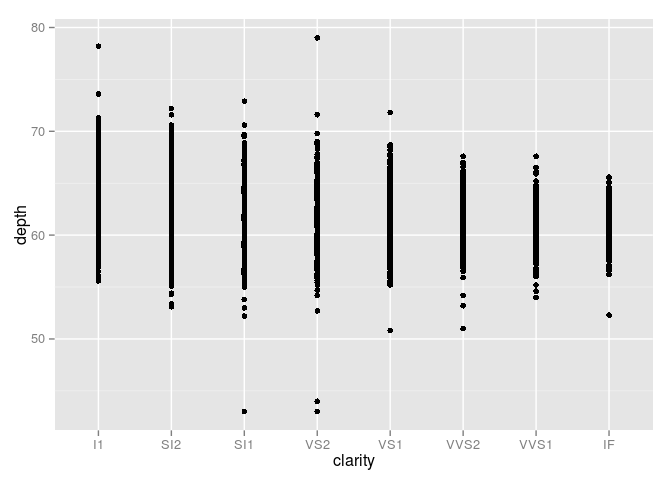Switching to a different data projection, like a boxplot, is as simple as “adding” a different geom_ to our original data structure:

p + geom_boxplot()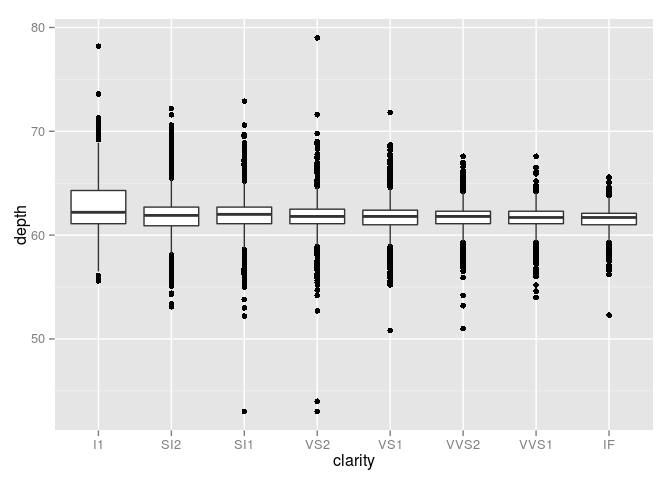As you can see, keeping with the spirit of the R environment, ggplot2 is designed to make it easy to rapidly iterate over different visualization and analysis approaches as you work interactively in the console. Simply take your underlying ggplot2 object describing data relationships and apply different visualization techniques to find one that suites your needs!

Single variable distributions

Above we saw two examples of how we visualize a quantitative variable grouped by a catagorical variable. When you begin exploring new datasets it is often quite useful to start with a focused look at the distribution of the data along each of your unique quantitative variables. Here are some examples:

# Visualize the distribution of the depth variable
p2 <- ggplot(diamonds, aes(depth))
# Histogram
p2 + geom_histogram()
## stat_bin: binwidth defaulted to range/30. Use 'binwidth = x' to adjust this.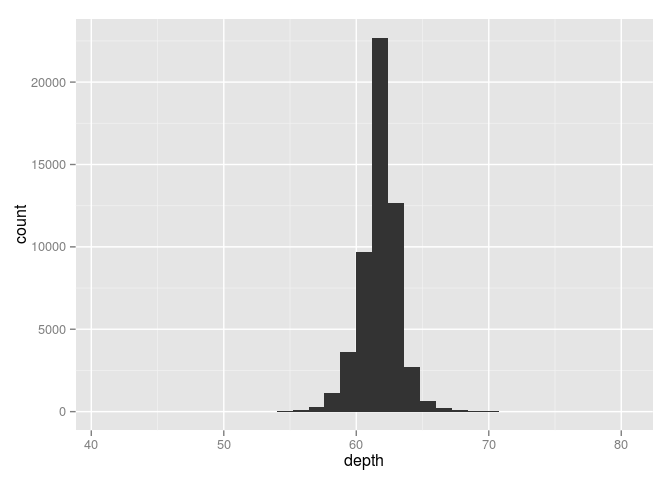# Density distribution
p2 + geom_density()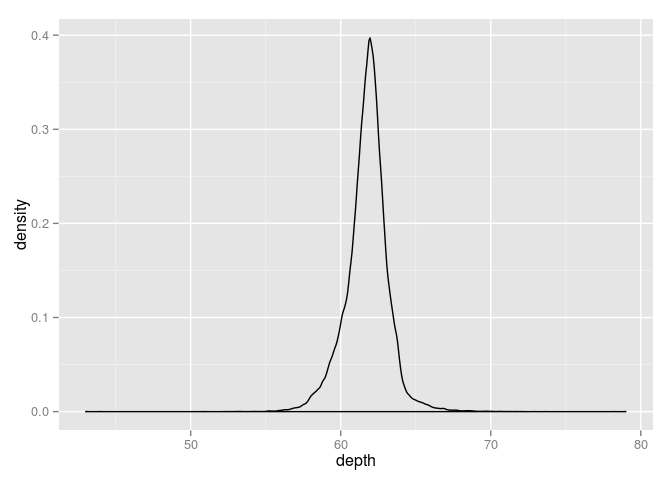Setting axis limits

There are other kinds of functions that can be applied to ggplot objects to tweak the visualization. For example, to set the axis limits on the above plot we can apply the xlim or ylim functions:

# Set x-axis limits
p2 + geom_density() + xlim( c(55, 65) )
## Warning: Removed 857 rows containing non-finite values (stat_density).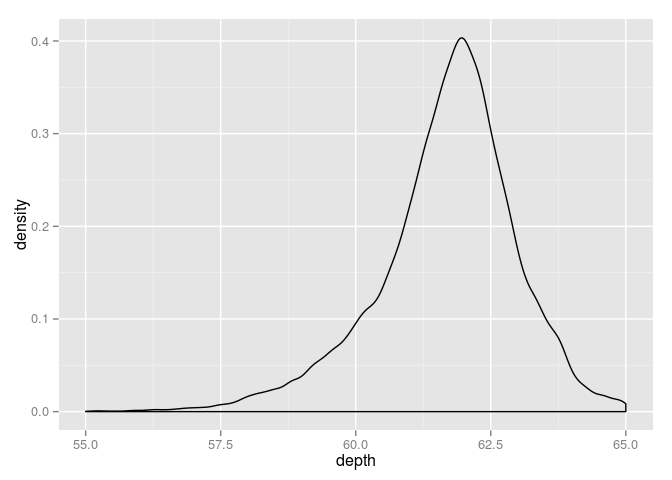When we run this code, we get a warning telling you that these limits have caused some data to be excluded from your plot. There are a number of useful sanity checks built into ggplot2 that warn you if your visualization parameters are running the risk of producing misleading results!

Two quantitative variables

Of course, we can also visualize the relationship between two quantitative variables using a scatter plot:

# Visualize depth as a function of price
p3 <- ggplot( diamonds, aes(price, depth) )
# Use points (scatter plot)
p3 + geom_point()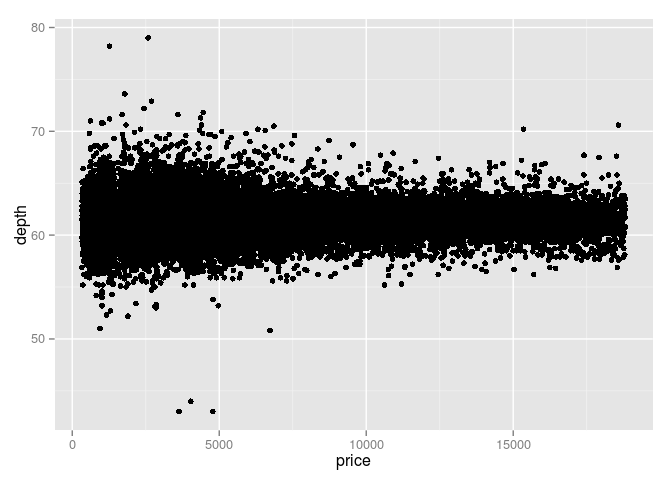Data grouping and secondary visualizations

In your aethetic mappings, you can describe more than just the initial structure of the data projection. For example, let’s say that we want to visualize the categorical variable clarity, when comparing price and depth:

# Visualize depth as a function of price; use color to visualize clarity label
p4 <- ggplot( diamonds, aes(price, depth, color = clarity) )
# Use points (scatter plot)
p4 + geom_point()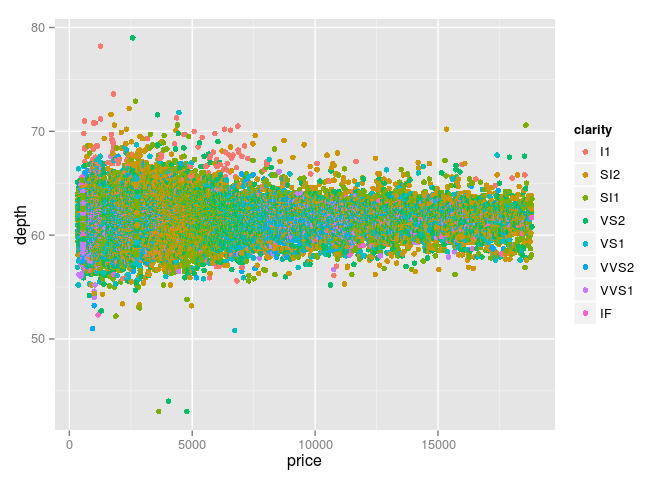For visualizations that use solid objects, you can also specify a fill argument:

p5 <- ggplot(diamonds, aes( price, fill = clarity) )
# Setting the alpha channel to 50% makes the density plots transparent
p5 + geom_density(alpha = 0.5)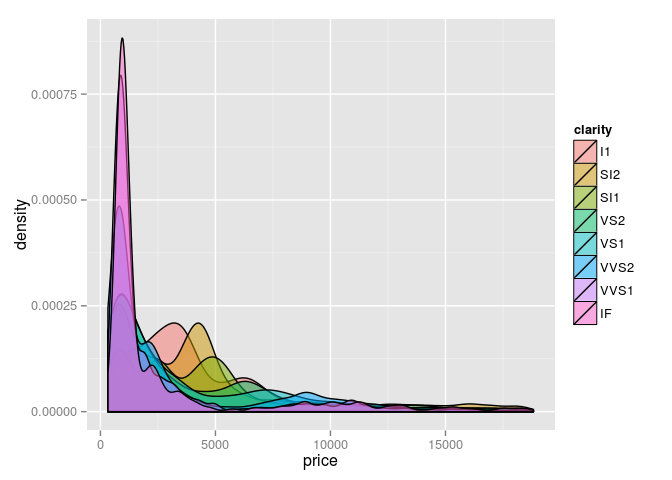There are many more options for visualizations schemes (geom_ functions), for mapping annotation variables onto additional visualization parameters (ex: color, fill, size, alpha), and for manipulating axes, labels and legends (ex: xlim, ylim, labs, coord_, scale_).

See the excellent ggplot2 documentation for the full list of functions with useful examples: docs.ggplot2.org.